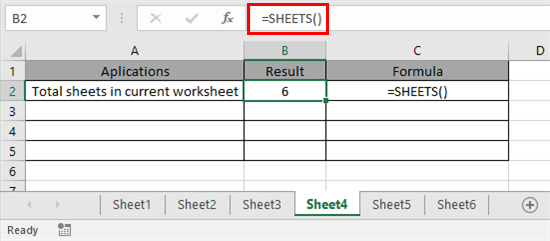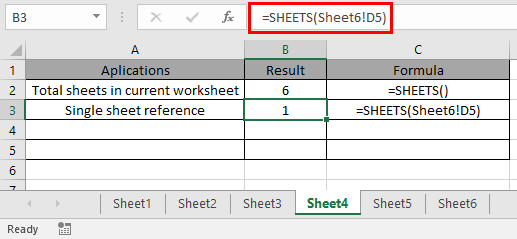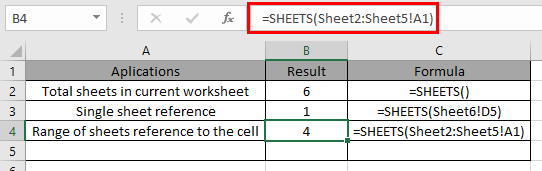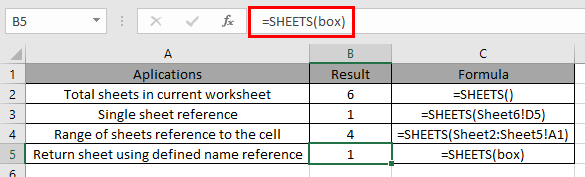# How to use the SHEETS function in Excel

In this article, we will learn about how to use the SHEETS function in Excel.
SHEETS function in excel is built-in reference function. This function returns a number, reference to input sheet indicated. SHEETS function can be used for following purposes in Excel.

1. The function returns the total number of sheets in the current worksheet.
2. The function returns the single sheet or Range of sheets reference.
3. The function returns the sheet reference using the defined name reference.

The SHEETS function is a cell reference function. SHEETS function in excel returns a number, referring the input sheet value

 =SHEETS ([reference])

Let’s understand this function using it in an example.
We will get to learn all the uses of the function. So we get through it on by one.

To get the total worksheets in the working or current excel file, use the function with empty parentheses.Here in the above snapshot, The function returns 6 as output as there are total 6 worksheets in the worksheet. The result of the formula in under Result column and Its formula in the adjacent column.

To get the single sheet reference, reference is provided to input argument of the function.See the above snapshot to view its application, Result & formula.

To get the range of sheet reference. See the below snapshot.Here a range of sheets are provided Sheet2:Sheet5. In the range there are 4 sheets. So the function returns 2 as output.

Use the named range to get the reference for the range of sheets via using the function.For the above result, we defined a table named range “box” as called its reference using the SHEETS function in the worksheet.

Here are some of the observational results about thr function.
Note:

1. The function includes all sheets ( hidden, visible or very hidden) including all other sheet types like macro, chart & dialog sheets.
2. The function returns the #REF! Error, if the input value is not a valid value.
3. Empty value in the SHEETS function, returns the total sheets in the current worksheet.
4. The function counts the 3D reference in the input.

Hope you understood how to use SHEETS function and referring cell in Excel. Explore more articles on Excel function here. Please feel free to state your query or feedback for the above article.

Related Articles
How to use the TYPE function
How to use the CELL function

## Comments

1.Is there a function that returns the worksheet name instead of the number of the worksheet?

•Hi Claire,

You can rename the worksheet name, just double click on sheet name and write the name as per your requirement then press enter on your keyboard, sheet name will get change.

Terms and Conditions of use

The applications/code on this site are distributed as is and without warranties or liability. In no event shall the owner of the copyrights, or the authors of the applications/code be liable for any loss of profit, any problems or any damage resulting from the use or evaluation of the applications/code.